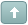Welcome Guest! You need to login or register to make posts.Error

# HOWTO: Make Monochrome Images

 Tamila #1 Posted : Wednesday, July 8, 2009 9:08:53 PM(UTC)Rank: Advanced MemberGroups: Joined: 3/9/2008(UTC)Posts: 554Was thanked: 1 time(s) in 1 post(s) Suppose, you have color RGB picture and you want to convert it to a red-white, yellow-white or blue-white (and so on) image analogous to standard grayscale one.As a solution I suggest the following algorithm for each pixel:Get the values of channels (R,G,B) in source bitmap.Calculate lightness.Compose HSL (Hue, Saturation, Lightness) color. Lightness of this pixel equals to the value calculated on the previous step. H and S values are defined as input values fixing monochrome color.Convert from HSL to RGB.Write down pixel into new bitmap.The following code represents the algorithm above. Take into account h and s parameters passed to this function forms monochrome color (in HSL format, L channel is omitted) we transform the image to.Code:``````Private Function HslToRgb(ByVal h As Double, ByVal s As Double, _ ByVal bmp As Aurigma.GraphicsMill.Bitmap) As Aurigma.GraphicsMill.Bitmap Dim bitmapData As Aurigma.GraphicsMill.BitmapData = bmp.LockBits() Dim stride As Integer = bitmapData.Stride Dim pointer As IntPtr = bitmapData.Scan0 Dim pixelSize As Integer = bitmapData.BitsPerPixel / 8 Dim newBmp As New Aurigma.GraphicsMill.Bitmap(bmp.Width, _ bmp.Height, Aurigma.GraphicsMill.PixelFormat.Format24bppRgb) Dim newBitmapData As Aurigma.GraphicsMill.BitmapData = newBmp.LockBits() Dim newPointer As IntPtr = newBitmapData.Scan0 Dim newStride As Integer = newBitmapData.Stride Dim colors(pixelSize - 1) As Byte Dim lChannel As Double Dim hChannel As Double Dim sChannel As Double Dim j As Integer = 0 For i As Integer = 0 To bitmapData.Height - 1 j = 0 Do While j < bmp.Width System.Runtime.InteropServices.Marshal.Copy(IntPtr.op_Explicit(pointer.ToInt32()+ _ i * stride + j * pixelSize), colors, 0, pixelSize) Dim blue As Byte = colors(0) Dim green As Byte = colors(1) Dim red As Byte = colors(2) Dim lightness As Byte = (30 * blue + 59 * green + 11 * red) / 100 lChannel = lightness / 255.0 hChannel = h sChannel = s Dim v, r, g, b As Double r = lChannel ' default to gray g = lChannel b = lChannel v = IIf((lChannel <= 0.5), (lChannel * (1.0 + sChannel)), _ (lChannel + sChannel - lChannel * sChannel)) If v > 0 Then Dim m, fract, vsf, mid1, mid2, sv As Double Dim sextant As Integer m = lChannel + lChannel - v sv = (v - m) / v hChannel *= 6.0 sextant = CInt(hChannel) fract = hChannel - sextant vsf = v * sv * fract mid1 = m + vsf mid2 = v - vsf Select Case (sextant) Case 0 r = v g = mid1 b = m Case 1 r = mid2 g = v b = m Case 2 r = m g = v b = mid1 Case 3 r = m g = mid2 b = v Case 4 r = mid1 g = m b = v Case 5 r = v g = m b = mid2 End Select End If colors(2) = Convert.ToByte(r * 255.0F) colors(1) = Convert.ToByte(g * 255.0F) colors(0) = Convert.ToByte(b * 255.0F) System.Runtime.InteropServices.Marshal.Copy(colors, 0, _ IntPtr.op_Explicit(newPointer.ToInt32() + i * newStride + j * pixelSize), pixelSize) j = j + 1 Loop Next i bmp.UnlockBits(bitmapData) newBmp.UnlockBits(newBitmapData) Return newBmp End Function`````` See also:HSL and HSVEdited by moderator Monday, May 28, 2012 8:31:38 PM(UTC)  | Reason: Not specifiedUsers browsing this topic
Forum Jump
You cannot post new topics in this forum.
You cannot reply to topics in this forum.
You cannot delete your posts in this forum.
You cannot edit your posts in this forum.
You cannot create polls in this forum.
You cannot vote in polls in this forum.